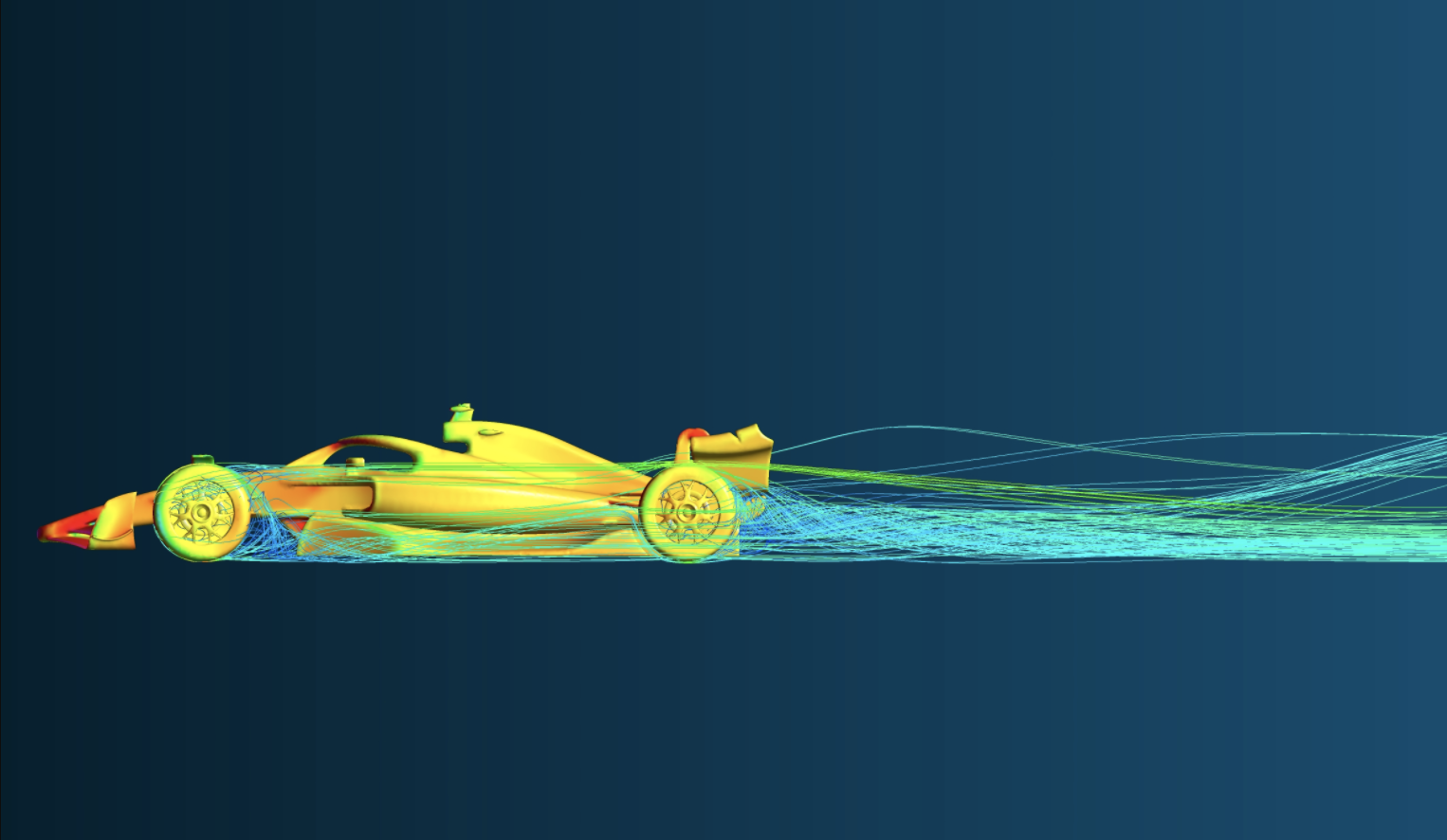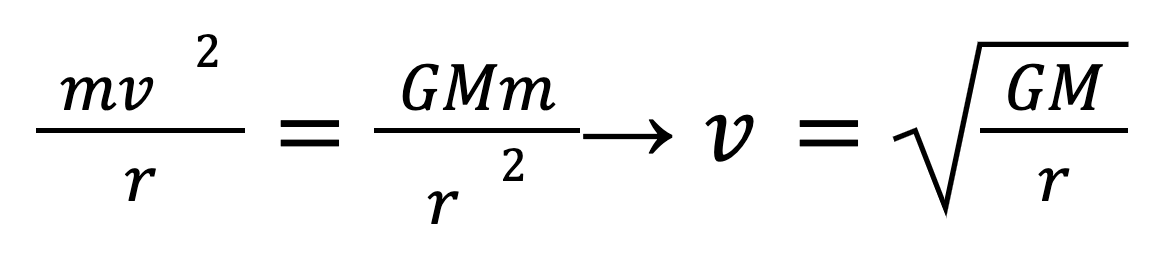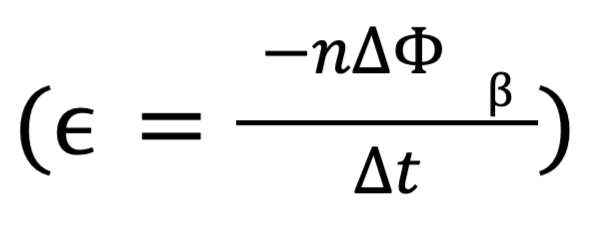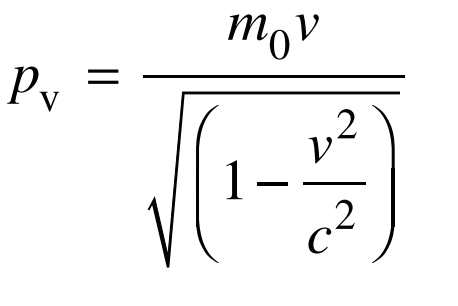NEW HORNSBY CAMPUS: Enrolments open for Term 4 2023! 🎉# HSC Physics: How To Answer Short and Long Form Answer QuestionsAlicia Pan 95 in Physics, 99.80 ATAR, All rounder

### What Does the HSC Physics Syllabus say About These Questions?

Looking at NESA’s Performance Band 6 Descriptions in the HSC Physics syllabus, we can get an idea of what’s expected of students in short and long answer writing questions. These responses require students to demonstrate an extensive knowledge and understanding of scientific concepts in the HSC Physics course and for them to communicate scientific understanding succinctly, logically and consistently. This means that markers are looking for how clearly students explain their understanding of certain concepts, including how they structure and present their response, whether they are using precise scientific terms and how well they apply their knowledge of content to answer questions involving unfamiliar situations. Students are also expected to respond to scientific problems using qualitative and quantitative data as well as apply processes to explain phenomena, make predictions and design solutions to scientific problems. This means that students should expect to be given a range of different types of questions, including but not limited to questions involving calculations, experimental procedures and stimulus diagrams.

### What Else is Expected in Physics Calculation Questions?

Aside from demonstrating knowledge of relevant calculations and getting the right answer, an important thing expected in calculation questions is to always round your final answer to the correct number of significant figures. You never know which questions in exams have a mark specifically allocated for correct rounding, so students should definitely ensure they always give their answers to the lowest significant figure.

## What are Teachers Looking for in your Responses?

### The Structure of your Responses

Good structure is really important in written answers and is something that teachers are always looking for, especially in longer responses. When you have to answer questions that are 7-9 marks, it can be quite easy to get carried away with trying to put down all the information you need to cover and so the real challenge is making sure you are succinct in answering the question. While seeing that a question is worth 9 marks may be quite overwhelming, remember that teachers aren’t looking at how much writing is on the page! Teachers and markers don’t want to see any ‘waffle’ so before starting any response, always ask yourself what you think each mark is allocated towards. In preparation for exams, it is helpful to look through the marking criteria of long response questions from your school exams and HSC Physics exams to get an idea of what teachers and markers are looking for and where marks are rewarded. Use the marks to help plan out what you want your structure to look like. Your plan can include the main points that you want to introduce, important definitions and any relevant formulas you want to include. By first organising your ideas, it will be easier to communicate your response in a logical manner, showing that you have a cohesive understanding of concepts.

(More tips on structure in next section)

### How you Format your Responses

While structure is how you organise your ideas in your answer, format is looking at how you present your answer on the page. It makes it much easier for teachers and markers to see key points underlined or boxed (signposting). For example, highlighting laws are extremely important. Part of this is also knowing important physics terms such as electromagnetic induction and where to use them effectively to help convey your points.

Depending on how you structure your response, make sure you have correct formatting. If you choose to do a long written response, break the question down into small headings and paragraphs. For example, for the development of the atomic model, break your response into Thomson, Rutherford, Bohr and Schrodinger. The effect of this is that markers can easily see if you have gone into enough depth, covered enough points and ultimately, your response will look far more appealing to read compared to a slab of text. Another way to format your answer, especially if you are writing about an experimental procedure, is to use numbered steps. Teachers will also be looking at the format of your tables (correct headings) and diagrams (labels).

### The Content Within your Responses

According to the HSC Physics syllabus, teachers are looking for how well students demonstrate an extensive knowledge and understanding of the Physics content while also looking at how well they can apply this knowledge to solve problems and answer unfamiliar questions. This means that rather than trying to write down everything you know about the topic as fast as you can, take some time to analyse the question and identify the most relevant content before answering.

### Make sure to Remember your Keywords!

A good thing to do before starting your response is to circle any keywords and key verbs in the question which you can consistently refer back to while writing to ensure you are answering the question. Remember that teachers are looking for how well you apply relevant knowledge to answer the question so it’s always good when they see that you have used the keywords throughout your response. Before your HSC Physics exams, it is really important that you read through NESA’s list of keywords along with the HSC Physics syllabus to understand exactly what each verb means so that you know how to approach questions.

### Correct Discussion of Theories and Their Relevance to the Question

Again, teachers are looking for how well you are able to apply your knowledge to solve problems so make sure that the theories you refer to are relevant to the question and help you prove your argument! Before starting your response, break down the question and jot down on the side of your page the theories or ideas you are planning to include. If you have quite a few that you are referencing, tick them off as you go so that you don’t forget any.

## What Sort Of Structure Do I Use to Answer These Questions?

Generally, for short answer questions, it helps to use a ‘cause and effect’ structure especially if the key verb is ‘explain’, ‘describe’ or other simple verbs. Make sure you explain the concepts logically from start to finish. If you mention any laws, always write the definitions and include any relevant formulas. For example, explaining how an EMF is induced:

Cause: A rate of change in magnetic flux is experienced by a moving conductor through a magnetic field.

Effect: By Faraday’s law (define and include equation), an EMF is induced inside the conductor and as such if the conductor has a closed loop, this causes a current to be induced in the direction given by Lenz’s law (define).

Depending on the question, there are quite a few ways you can approach structuring your long responses.

Sometimes you may find that it is helpful to incorporate diagrams into your response structure. Questions that require you to talk about scientific experiments are often time-consuming to explain in words as it might be difficult to describe the specific details of the experiment and the apparatus. For example, if you have to describe Young’s double-slit experiment, this is where diagrams come in handy and can save you a lot of time! (More tips on diagrams in later section)

Another way you can structure your response is by using a table which is a great way to answer a comparison question. For example, questions may ask you to compare GEO and LEO satellites, DC and AC generators or Newton and Huygen’s model of light. Since you have to address the similarities and differences using different sub-topics, using a table saves you time from having to link anything together!

A good way to practice structuring written answer questions is to just make plans! Instead of writing out a full response, go through past exam questions and write out a plan, using the number of marks to guide you. Map out your structure by jotting down the main points you would include, any relevant theories, laws, formulas and diagrams you might want to use.

## Exemplar Questions for HSC Physics

Q1. Why does the mass of a body play no role in determining its orbital speed around a planet? (2 marks)

Orbital speed is calculated by equating force due to gravity with the centripetal force required to maintain circular motion:(independent of mass m)

Therefore mass of body plays no role in determining its orbital speed.

Q2. Alex the olympian throws a discus across a field. She releases the discus at a velocity of 18 m/s at an angle of 30 degrees. Explaining the physics behind your answer, find the final horizontal velocity of the discus. (3 marks)

Once released, the discus is a projectile and therefore will undergo projectile motion. By definition, the only force the discus will experience is gravity directly downwards. Since the force only acts in the vertical plane, there is no change to the horizontal velocity through the motion.

Therefore:

final horizontal velocity = initial horizontal velocity

= 18 cos(30)

= 16 m/s

Module 5 is probably the most maths-based module so the written responses may involve calculations and will require you to show your working out. For circular motion questions, drawing free body diagrams might make explanations easier. The most content-heavy part of Module 5 is the ‘Motion in Gravitational Fields’ topic questions might get you to write about Kepler’s laws, different types of satellites or energy changes between orbits.

### Module 6: Electromagnetism

Q1. Explain how a magnet falling down a copper tube experiences a resistive force. (3 marks)

A moving magnet imposes a changing magnetic flux through the walls of the copper tube. By Faraday’s Law, this changing flux induces an emf:and by Lenz’s Law, this emf induces a current to oppose this change in magnetic flux. This induced current (eddy current) creates a magnetic field that opposes that of the magnet, and therefore produces a repelling force that resists the falling motion of the magnet.

Q2. Explain how a current is induced in the secondary coil of a transformer, and why it only works with AC but not DC circuits. (2 marks)

An AC input voltage is applied to a primary coil of a transformer around a laminated soft-iron core, which creates a changing magnetic field around the secondary coil. By Faraday’s Law, this changing magnetic flux induces an emf in the secondary coil, which thus produces a current when connected to a circuit. AC must be used in order to generate the changing magnetic flux required for induction (DC is constant).

Q3. 2021 HSC Physics Exam Past Paper Question 31 (7 marks)

When the switch on Cart 1 is closed, a constant direct current will flow through the solenoid which will be observed on G1. Using the right hand grip rule, this current will create a magnetic field in the solenoid with a south pole facing Cart 2. Cart 2 experiences an increase in magnetic field, thus a rate of change of magnetic flux which will induce an emf by Faraday’s law, given by:Since the circuit on Cart 2 is complete, an induced current will flow through its solenoid. According to Lenz’s law, the induced current is in such a direction that creates a magnetic field which opposes the initial change in flux. Thus, the solenoid on Cart 2 will create magnetic flux towards the right, hence forming a south pole facing Cart 1. This induced current will cause a deflection in G2 in the opposite direction to the current in G1.

Since the south poles formed are facing each other, both carts will experience an equal and opposite force of magnetic repulsion causing them to move away from each other. Since Cart 1 is double the mass of Cart 2 and the force of repulsion is equal in magnitude, according to Newton’s Second Law (F=ma), the acceleration of Cart 1 will be half the magnitude of Cart 2’s acceleration.

Make sure you have a really good understanding of electromagnetic induction as most questions will require you to use Faraday and Lenz’s law. Common Mod 6 questions also involve motors, generators or transformers so make sure you have an in-depth understanding of how these work, as well as their advantages, limitations and real-world application.

### Module 7: The Nature Of Light

Q1. Describe an experiment to justify the belief that light is a transverse wave. Clearly state why the described behaviour of light supports a transverse wave model of light. (3 marks)

Unpolarised light was passed through a polariser with a vertical axis. This polarised light was then passed to a second polarising filter (an analyser). As the analyser was rotated through 90°, the light intensity was observed.

Since transverse light waves have a plane of oscillation, the polariser only allows light waves with vertical axes of oscillation to pass through, while waves in the horizontal plane cannot pass through. When the analyser’s plane of polarisation is horizontal, no polarised light can pass through. The light intensity will increase as the analyser is rotated 90°. Once its plane

of polarisation is aligned with the first polariser, all the polarised light can pass through it. Polarisation was a known property of transverse waves as longitudinal waves cannot be polarised and will pass through both horizontal and vertical planes, thus this experiment supports light being a transverse wave model of light.

Q2. Einstein’s relativistic momentum equation is:Use this equation to explain why there is a limitation on the maximum velocity of a particle. (3 marks)

As the velocity of a particle approaches the speed of light, v approaches c and the denominator in the equation approaches 0, thus momentum would be infinite. This means that accelerating an object up to v=c would require infinite energy which is not possible as it would violate the law of conservation of energy which states that energy cannot be created or destroyed, only transferred or transformed. Hence, there is a limitation on the maximum velocity of a particle as the speed of light cannot be exceeded.

Q3. 2021 HSC Physics Exam Past Paper Question Sample Answers 33 (9 marks)

Experiment A supports the wave model of light as it demonstrates light exhibiting wave phenomena such as diffraction and interference. When the light passes through the double slits, it diffracts through each slit causing wavefronts to bend as they pass through. The wavefronts then superimpose causing interference. Constructive interference causes bright bands to form while destructive interference causes dark bands to form, as seen in the interference pattern on the screen.

dsinθ = mλ

y=ltanθ

since θ is small, we can assume sinθ=tanθ=θ

θ=mλ/d

θ= y/L

y=mLλ/d

Therefore we can calculate the spacing between each adjacent band.

When m=1

y = (1×3.0×400×10^-9) / (5.0×10^-5)

= 0.024 m

Experiment B supports the particle model of light as it shows the photoelectric effect. The photoelectric effect assumes light exists in quantised, packets of energies called photons. These photons carry energy given by E = hf = hc / λ. Therefore, the energy of light with wavelength 400 nm is:

E = hc / λ = (6.626x10^-34×3.00×10^8) / (400×10^-9)

= 4.97×10^-19 J

This energy of each photon explains why the light only ejects electrons from Calcium and not Nickel. Photons interact with electrons in a one-to-one ratio and only eject them when the energy of the photon is greater than the work function of the metal, which is the minimum energy required to liberate electrons. The maximum kinetic energy of the electrons is given by KE = hf - Φ. Thus, if energy (hf) is not greater than the work function (Φ), the electron will not have the kinetic energy to escape the metal surface. Since Nickel has a work function of 8.25 × 10^–19 J, the photons do not have sufficient energy to eject electrons hence no photoelectrons are observed. However, Calcium has a work function of 4.60 × 10^–19 and thus photons have enough energy to eject electrons. Using KE = hf - Φ, the kinetic energy of photoelectrons emitted from calcium can be calculated:

KE = (4.97 × 10^-19) – (4.60 × 10^–19)

= 3.70 × 10^-20 J

Module 7 is where it starts to get quite content-heavy and involves a lot of Physics history. Common Mod 7 questions will require you to write about the history of the speed of light, the development of the model of light as well as the experiments relevant to the discovery of light properties. Make sure you understand how all the main discoveries led to the development of new theories and all the supporting experimental evidence.

### Module 8: From the Universe To The Atom

Q1. Outline the characteristics of a main sequence star. (3 marks)

Main sequence stars like our sun lie in the centre of the Herztsprung-Russel diagram, and are the primary stage of a stars life cycle. They are powered by the fusion of hydrogen into helium via the Proton-Proton chain, and are composed of mostly hydrogen and helium, with trace amounts of other elements depending on what generation star they are. They are held together by a delicate balance between gravity compressing them inwards and radiative pressure pushing them outwards, and form red giants when they age sufficiently. Aside from these key characteristics, all other aspects of the star (size, luminosity, temperature and lifetime) are all determined by the mass of the star and thus vary across main sequence stars.

Q2. Analyse the role of Max Planck in the development of the Bohr model of the atom. (3 marks)

Planck’s ideas around quantisation formed the basis of Bohr’s model. The concept that light could exist in discrete states with a specific amount of energy acted as a precursor to Bohr’s description of electron stationary states with quantised energy levels.

Furthermore, this led to the idea of quantised angular momentum in these stationary states, as each electron state is at a specific distance from the nuclei with a specific amount of momentum and energy.

Finally, Planck’s equation E = hf was key to Bohr’s third postulate, which explained that electrons transitioned between states by absorbing and releasing electromagnetic radiation. The frequency of this electromagnetic radiation therefore directly relates to the energy lost or gained by the electron, and this accounts for the existence of emission and absorption spectra.

Q3. 2021 HSC Physics Exam Past Paper Question 29 (5 marks)

Bohr proposed that the nucleus of the atom was composed of protons and neutrons, with electrons orbiting the nucleus in quantised energy levels. Differing from Bohr’s atomic model, de Broglie proposed the concept of wave-particle duality which suggests that moving particles have an associated wavelength given by λ = h / mv and that moving electrons set up stable standing waves around the nucleus. Schrodinger’s model of the atom assumes the electron as a wave and describes regions of space where electrons are most likely to be found. Contrasting to Bohr and de Broglie’s atomic model where electron orbits are pre-determined, Schrodinger’s model is probabilistic.

Like Mod 7, Mod 8 is also pretty content-heavy. Mod 8 also involves quite a lot of history which means a lot of the questions will require you to demonstrate your understanding of scientific developments (For example, the development of the atomic model). It’s very likely that you will get a Mod 8 long response question so it’s important to practice how you would structure these responses.

### How Can I Use Diagrams To Enhance My Answer?

Using a diagram to enhance your answer is sometimes hard because students often think that including any diagram is sufficient. What’s key is knowing what and when diagrams aid answers. E.g)  A really good time to use a diagram is in a long response for atomic models to help explain the structure of any of the atomic models that would otherwise, be a little more difficult to explain with words. Another thing I would say is helpful is including a short description or label to help explain what the model depicts e.g) in figure 1.01 Rutherford’s atomic model is shown.

### So what next?

Some of the Year 11 concepts and skills are very similar to the ones you use in Year 12. However, in Year 12 you will be applying these skills to solve much more complex problems. There is also a lot of HSC Physics content that is very different from Year 11, you’ll learn about how physicists discovered the nature of light, atomic structure and the origins of our universe.

## Maximise Your Chances Of Coming First At School

Trial any Project Academy course for 3 weeks.

NSW's Top 1% Tutors

Unlimited Tutorials

NSW's Most Effective Courses

## Maximise Your Chances Of Coming First At School

Trial any Project Academy course for 3 weeks.

NSW's Top 1% Tutors

Unlimited Tutorials

NSW's Most Effective Courses